# Circular Singly Linked List | Insertion

• Difficulty Level : Easy
• Last Updated : 24 Jun, 2022

We have discussed Singly and Circular Linked List in the following post:

Why Circular? In a singly linked list, for accessing any node of the linked list, we start traversing from the first node. If we are at any node in the middle of the list, then it is not possible to access nodes that precede the given node. This problem can be solved by slightly altering the structure of a singly linked list. In a singly linked list, the next part (pointer to next node) is NULL. If we utilize this link to point to the first node, then we can reach the preceding nodes. Refer to this for more advantages of circular linked lists.
The structure thus formed is a circular singly linked list and looks like this: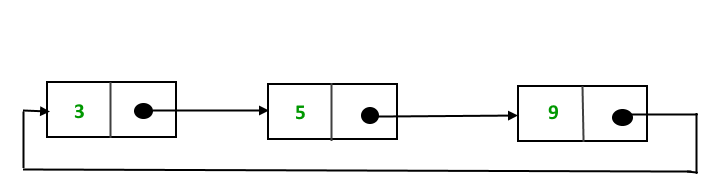In this post, the implementation and insertion of a node in a Circular Linked List using a singly linked list are explained.

Implementation
To implement a circular singly linked list, we take an external pointer that points to the last node of the list. If we have a pointer last pointing to the last node, then last -> next will point to the first node.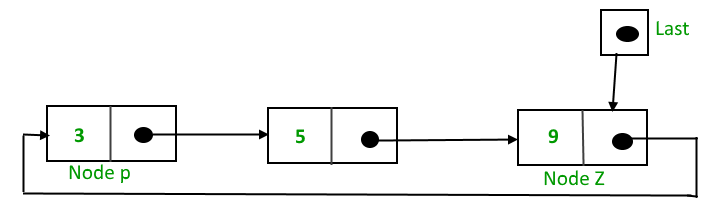The pointer last points to node Z and last -> next points to node P.

Why have we taken a pointer that points to the last node instead of the first node?
For the insertion of a node at the beginning, we need to traverse the whole list. Also, for insertion at the end, the whole list has to be traversed. If instead of start pointer, we take a pointer to the last node, then in both cases there won’t be any need to traverse the whole list. So insertion at the beginning or at the end takes constant time, irrespective of the length of the list.Insertion
A node can be added in three ways:

• Insertion in an empty list
• Insertion at the beginning of the list
• Insertion at the end of the list
• Insertion in between the nodes

Insertion in an empty List
Initially, when the list is empty, the last pointer will be NULL.After inserting node T,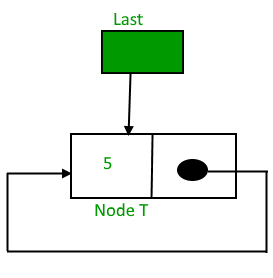After insertion, T is the last node, so the pointer last points to node T. And Node T is the first and the last node, so T points to itself.
Function to insert a node into an empty list,

## C++

 `struct` `Node *addToEmpty(``struct` `Node *last, ``int` `data) ` `{ ` `    ``// This function is only for empty list ` `    ``if` `(last != NULL) ` `      ``return` `last; ` ` `  `    ``// Creating a node dynamically. ` `    ``struct` `Node *temp = ` `          ``(``struct` `Node*)``malloc``(``sizeof``(``struct` `Node)); ` ` `  `    ``// Assigning the data. ` `    ``temp -> data = data; ` `    ``last = temp; ` `    ``// Note : list was empty. We link single node ` `    ``// to itself. ` `    ``temp -> next = last; ` ` `  `    ``return` `last; ` `} `

## Java

 `static` `Node addToEmpty(Node last, ``int` `data) ` `{ ` `    ``// This function is only for empty list ` `    ``if` `(last != ``null``) ` `      ``return` `last; ` ` `  `    ``// Creating a node dynamically. ` `    ``Node temp = ``new` `Node(); ` ` `  `    ``// Assigning the data. ` `    ``temp.data = data; ` `    ``last = temp; ` `    ``// Note : list was empty. We link single node ` `    ``// to itself. ` `    ``temp.next = last; ` ` `  `    ``return` `last; ` `} ` ` `  `// This code is contributed by gauravrajput1  `

## Python3

 `# This function is only for empty list ` `   ``def` `addToEmpty(``self``, data): `   `       ``if` `(``self``.last !``=` `None``): ` `           ``return` `self``.last `   `       ``# Creating the newnode temp ` `       ``temp ``=` `Node(data) ` `       ``self``.last ``=` `temp `   `       ``# Creating the link ` `       ``self``.last.``next` `=` `self``.last ` `       ``return` `self``.last ` ` ``# this code is contributed by shivanisinghss2110`

## C#

 `static` `Node addToEmpty(Node last, ``int` `data) ` `{ ` `    ``// This function is only for empty list ` `    ``if` `(last != ``null``) ` `      ``return` `last; ` ` `  `    ``// Creating a node dynamically. ` `    ``Node temp = ` `          ``new` `Node(); ` ` `  `    ``// Assigning the data. ` `    ``temp.data = data; ` `    ``last = temp; ` `    ``// Note : list was empty. We link single node ` `    ``// to itself. ` `    ``temp.next = last; ` ` `  `    ``return` `last; ` `} ` ` `  ` `  `// This code contributed by umadevi9616 `

## Javascript

 ` `

Time Complexity: O(1)

As we have to perform constant number of operations.

Auxiliary Space: O(1)

As constant extra space is used.

•
Insertion at the beginning of the list
To insert a node at the beginning of the list, follow these steps:
1. Create a node, say T.
2. Make T -> next = last -> next.
3. last -> next = T.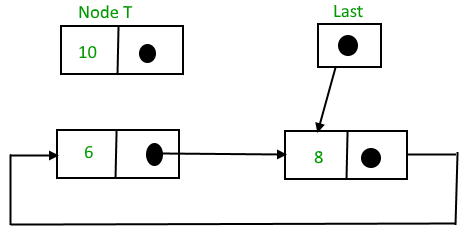After insertion,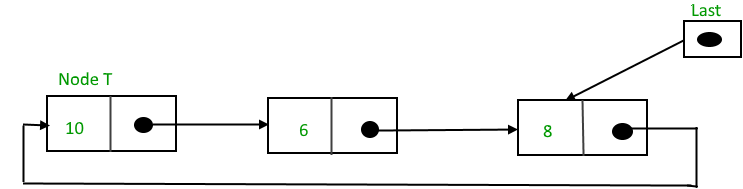Function to insert nodes at the beginning of the list,

## C++

 `struct` `Node *addBegin(``struct` `Node *last, ``int` `data) ` `{ ` `  ``if` `(last == NULL) ` `     ``return` `addToEmpty(last, data); ` ` `  `  ``// Creating a node dynamically. ` `  ``struct` `Node *temp ` `        ``= (``struct` `Node *)``malloc``(``sizeof``(``struct` `Node)); ` `   `  `  ``// Assigning the data. ` `  ``temp -> data = data; ` ` `  `  ``// Adjusting the links. ` `  ``temp -> next = last -> next; ` `  ``last -> next = temp; ` `   `  `  ``return` `last; ` `} `

## Java

 `static` `Node addBegin(Node last, ``int` `data) ` `{ ` `    ``if` `(last == ``null``) ` `        ``return` `addToEmpty(last, data); ` `   `  `      ``// Creating a node dynamically ` `    ``Node temp = ``new` `Node(); ` `      `  `      ``// Assigning the data ` `    ``temp.data = data; ` `   `  `      ``// Adjusting the links ` `    ``temp.next = last.next; ` `    ``last.next = temp; ` `  `  `    ``return` `last; ` `} ` ` `  `// This code is contributed by rutvik_56`

## Python3

 `def` `addBegin(``self``, data): `   `       ``if` `(``self``.last ``=``=` `None``): ` `           ``return` `self``.addToEmpty(data) `   `       ``temp ``=` `Node(data) ` `       ``temp.``next` `=` `self``.last.``next` `       ``self``.last.``next` `=` `temp `   `       ``return` `self``.last ` ` ``# this code is contributed by shivanisinghss2110`

## C#

 `static` `Node addBegin(Node last, ``int` `data) ` `{ ` `    ``if` `(last == ``null``) ` `        ``return` `addToEmpty(last, data); ` ` `  `    ``// Creating a node dynamically ` `    ``Node temp = ``new` `Node(); ` ` `  `    ``// Assigning the data ` `    ``temp.data = data; ` ` `  `    ``// Adjusting the links ` `    ``temp.next = last.next; ` `    ``last.next = temp; ` ` `  `    ``return` `last; ` `} ` ` `  `// This code is contributed by Pratham76`

## Javascript

 ``

Time complexity : O(1) to insert node at beginning no need to traverse list it takes constant time

Space Complexity : O(1)

•
Insertion at the end of the list
To insert a node at the end of the list, follow these steps:
1. Create a node, say T.
2. Make T -> next = last -> next;
3. last -> next = T.
4. last = T.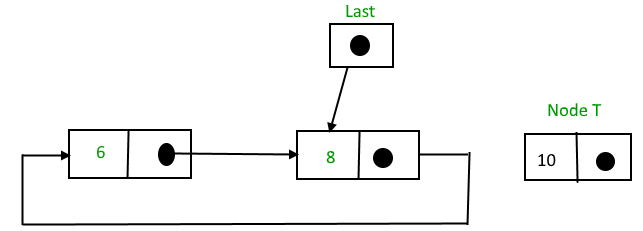After insertion,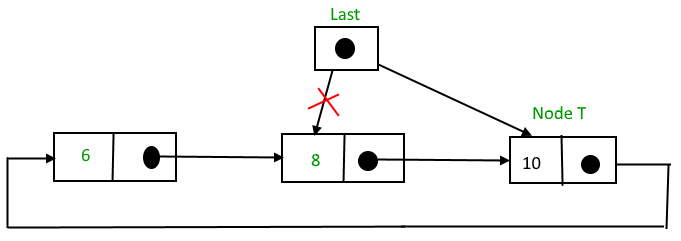Function to insert a node at the end of the List

## C++

 `struct` `Node *addEnd(``struct` `Node *last, ``int` `data) ` `{ ` `  ``if` `(last == NULL) ` `     ``return` `addToEmpty(last, data); ` ` `  `  ``// Creating a node dynamically. ` `  ``struct` `Node *temp =  ` `        ``(``struct` `Node *)``malloc``(``sizeof``(``struct` `Node)); ` `   `  `  ``// Assigning the data. ` `  ``temp -> data = data; ` ` `  `  ``// Adjusting the links. ` `  ``temp -> next = last -> next; ` `  ``last -> next = temp; ` `  ``last = temp; ` `   `  `  ``return` `last; ` `} `

## Java

 `   ``static` `Node addEnd(Node last, ``int` `data) ` `   ``{ ` `   ``if` `(last == ``null``) ` `       ``return` `addToEmpty(last, data); ` `        `  `// Creating a node dynamically.     ` `   ``Node temp = ``new` `Node(); ` ` `  `// Assigning the data. ` `   ``temp.data = data; ` `    `  `// Adjusting the links.     ` `   ``temp.next = last.next; ` `   ``last.next = temp; ` `   ``last = temp; `   `   ``return` `last; ` `   ``} ` `    `  `// This code is contributed by shivanisinghss2110        `

## Python3

 `def` `addEnd(``self``, data): ` ` `  `        ``if` `(``self``.last ``=``=` `None``): ` `            ``return` `self``.addToEmpty(data) ` ` ``# Assigning the data.  ` `        ``temp ``=` `Node(data) ` `   `  `  ``# Adjusting the links. ` `        ``temp.``next` `=` `self``.last.``next` `        ``self``.last.``next` `=` `temp ` `        ``self``.last ``=` `temp ` ` `  `        ``return` `self``.last ` `       `  `   ``# This code is contributed by shivanisinghss2110`

## C#

 `static` `Node addEnd(Node last, ``int` `data) ` `{ ` `    ``if` `(last == ``null``) ` `        ``return` `addToEmpty(last, data); ` `     `  `    ``// Creating a node dynamically.   ` `    ``Node temp = ``new` `Node(); ` `  `  `    ``// Assigning the data.  ` `    ``temp.data = data; ` `   `  `    ``// Adjusting the links.   ` `    ``temp.next = last.next; ` `    ``last.next = temp; ` `    ``last = temp; ` `  `  `    ``return` `last; ` `} ` ` `  `// This code is contributed by shivanisinghss2110`

## Javascript

 ``

Complexity analysis :

Time Complexity : O(1) to insert a node at the end of the list. No need to traverse the list as we are utilizing the last pointer, hence it takes constant time.

Space Complexity : O(1)

• Insertion in between the nodes
To insert a node in between the two nodes, follow these steps:
1. Create a node, say T.
2. Search for the node after which T needs to be inserted, say that node is P.
3. Make T -> next = P -> next;
4. P -> next = T.
Suppose 12 needs to be inserted after the node has the value 10,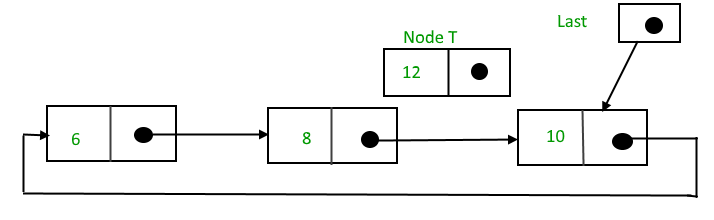After searching and insertion,Function to insert a node at the specified position of the List,

## C++

 `struct` `Node *addAfter(``struct` `Node *last, ``int` `data, ``int` `item) ` `{ ` `    ``if` `(last == NULL) ` `       ``return` `NULL; ` ` `  `    ``struct` `Node *temp, *p; ` `    ``p = last -> next; ` ` `  `    ``// Searching the item. ` `    ``do` `    ``{ ` `        ``if` `(p ->data == item) ` `        ``{ ` `            ``// Creating a node dynamically. ` `            ``temp = (``struct` `Node *)``malloc``(``sizeof``(``struct` `Node)); ` ` `  `            ``// Assigning the data. ` `            ``temp -> data = data; ` ` `  `            ``// Adjusting the links. ` `            ``temp -> next = p -> next; ` ` `  `            ``// Adding newly allocated node after p. ` `            ``p -> next = temp; ` ` `  `            ``// Checking for the last node. ` `            ``if` `(p == last) ` `                ``last = temp; ` ` `  `            ``return` `last; ` `        ``} ` `        ``p = p -> next; ` `    ``} ``while` `(p != last -> next); ` ` `  `    ``cout << item << ``" not present in the list."` `<< endl; ` `    ``return` `last; ` `} `

## Java

 `static` `Node addAfter(Node last, ``int` `data, ``int` `item) ` `{ ` `    ``if` `(last == ``null``) ` `        ``return` `null``; ` ` `  `    ``Node temp, p; ` `    ``p = last.next; ` `    ``do` `    ``{ ` `        ``if` `(p.data == item) ` `        ``{ ` `            ``temp = ``new` `Node(); ` `            ``temp.data = data; ` `            ``temp.next = p.next; ` `            ``p.next = temp; ` ` `  `            ``if` `(p == last) ` `                ``last = temp; ` `            ``return` `last; ` `        ``} ` `        ``p = p.next; ` `    ``} ``while``(p != last.next); ` ` `  `    ``System.out.println(item + ``" not present in the list."``); ` `    ``return` `last; ` ` `  `} ` ` `  `// This code is contributed by shivanisinghss2110`

## Python3

 `def` `addAfter(``self``, data, item): ` ` `  `        ``if` `(``self``.last ``=``=` `None``): ` `            ``return` `None` ` `  `        ``temp ``=` `Node(data) ` `        ``p ``=` `self``.last.``next` `        ``while` `p: ` `            ``if` `(p.data ``=``=` `item): ` `                ``temp.``next` `=` `p.``next` `                ``p.``next` `=` `temp ` ` `  `                ``if` `(p ``=``=` `self``.last): ` `                    ``self``.last ``=` `temp ` `                    ``return` `self``.last ` `                ``else``: ` `                    ``return` `self``.last ` `            ``p ``=` `p.``next` `            ``if` `(p ``=``=` `self``.last.``next``): ` `                ``print``(item, ``"not present in the list"``) ` `                ``break` `                 `  `# This code is contributed by shivanisinghss2110`

## C#

 `static` `Node addAfter(Node last, ``int` `data, ``int` `item) ` `{ ` `    ``if` `(last == ``null``) ` `        ``return` `null``; ` `  `  `    ``Node temp, p; ` `    ``p = last.next; ` `    ``do` `    ``{ ` `        ``if` `(p.data == item) ` `        ``{ ` `            ``temp = ``new` `Node(); ` `            ``temp.data = data; ` `            ``temp.next = p.next; ` `            ``p.next = temp; ` `  `  `            ``if` `(p == last) ` `                ``last = temp; ` `            ``return` `last; ` `        ``} ` `        ``p = p.next; ` `    ``} ``while``(p != last.next); ` `     `  `      ``Console.WriteLine(item + ``" not present in the list."``); ` `    ``return` `last; ` `  `  `} ` ` `  `// This code is contributed by shivanisinghss2110`

## Javascript

 ``

Complexity analysis :

Time Complexity : O(N)

Auxiliary Space : O(1)

The following is a complete program that uses all of the above methods to create a circular singly linked list.

Code block

Output:

`2 4 6 8 10 12`

Complexity Analysis :

Time Complexity: O(N), for above code we need O(1) constant time to insert node at first position and to insert node at tail or end and in between list it will take O(N). so we ignore the constants so overall time complexity is O(N) for insertion operation in circular linked list.

Auxiliary Space: O(1), as we are not using any extra space.

This article is contributed by Anuj Chauhan. If you like GeeksforGeeks and would like to contribute, you can also write an article using write.geeksforgeeks.org or mail your article to review-team@geeksforgeeks.org. See your article appearing on the GeeksforGeeks main page and help other Geeks.